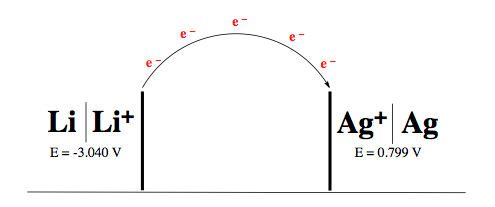Clutch Prep is now a part of Pearson
Ch.13 - Fundamentals of ElectrochemistryWorksheetSee all chapters

# Standard Potentials

See all sections
Sections
Basic Concepts
Electrochemical Cells
Standard Potentials
Nernst Equation
Standard Cell Potential & the Equilibrium Constant
###### Calculating Standard Potential

Concept #1: Voltage (E) represents the amount of work done in an electrochemical cell as electrons travel from one electrode to another.

Example #1: Determine the electric potential that results from the given galvanic cell.###### Standard Potential Calculations

Example #2: Use the standard half-cell potentials listed below to calculate the standard cell potential for the following reaction occurring in an electrochemical cell at 25°C. Assume the concentrations have approached unity.

3 Cl (g) + 2 Fe (s) ⇌ 6 Cl (aq) + 2 Fe3+ (aq)

Half Reactions:

Cl2 (g) + 2 e  → 2 Cl (aq)                      E° = + 1.396 V
Fe3+ (aq) + 3 e  →  Fe (s)                         E° = – 0.040 V

Example #3: For the a voltaic cell with the overall reaction:

Zn (s) + Cu2+ (aq) ⇌ Zn2+ (aq) + Cu (s)               E°cell = 1.10 V

Given that the standard reduction potential of Zn2+ to Zn (s) is – 0.762 V, calculate the standard reduction potential for:

Cu2+ (aq) + 2 e  →  Cu (s)